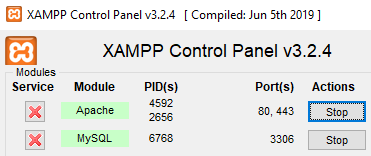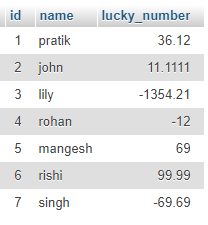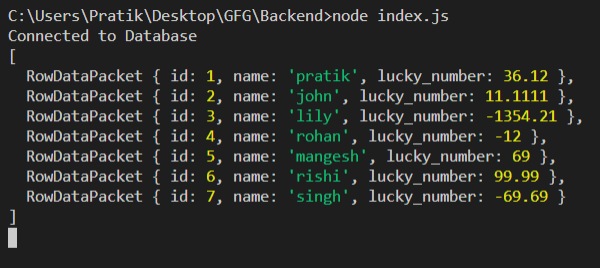Open in App
Not now

# How to save connection result in a variable in Node.js ?

• Last Updated : 07 Apr, 2021

We are going to use the query function in MySQL library in node.js that will return our output as expected. Using this approach, we can save connection result in a variable in Node.js.

Setting up environment and Execution:

Step 1: Initialize node project using the following command.

`npm init`

Step 2: Turn on MySQL DatabaseStep 3: After creating the NodeJS application, Install the mysql module using the following command.

`npm install mysql`

Database Table: Our sample gfg_table database with look like this.Step 4: Create an index.js file with the following code.

## index.js

 `const mysql = require(``"mysql"``);`` ` `var` `db_con = mysql.createConnection({``    ``host: ``"localhost"``,``    ``user: ``"root"``,``    ``password: ``""``,``    ``database: ``"gfg_db"``});`` ` `let output;`` ` `const setOutput = (rows) => {``    ``output = rows;``    ``console.log(output);``}`` ` `db_con.connect(async(err) => {``    ``if` `(err) {``        ``console.log(``"Database Connection Failed !!!"``, err);``        ``return``;``    ``}`` ` `    ``console.log(``"Connected to Database"``);`` ` `    ``let query = ``'SELECT * FROM users'``;``    ``db_con.query(query, (err, rows) => {``        ``if` `(err) {``            ``console.log(``"internal error"``, err);``            ``return``;``        ``}``         ` `        ``// This is the important function``        ``setOutput(rows);``    ``});``});`

Step 5: Run the index.js file using the following command.

`node index.js`

Output:My Personal Notes arrow_drop_up